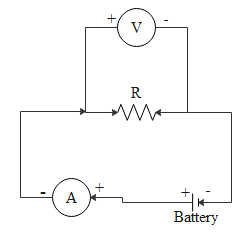Courses
Courses for Kids
Free study material
Offline Centres
MoreLast updated date: 25th Nov 2023
Total views: 278.1k
Views today: 4.78k

# Draw a neat diagram of Electrical circuit for studying ohm’s law.Verified
278.1k+ views
Hint: First of all to draw the electrical circuit we have to know what is ohm’s law. Ohm’s can be stated as, at constant temperature the voltage is directly proportional to the current flowing through the conductor. And the resistance is here the proportionality constant.
Formula used:
V=IR
Where, V is the potential difference
I is the current through the conductor
R a constant known as resistance

Complete step-by-step solution:
For this we consider an electric circuit,Thus Ohm’s law can be stated as at constant temperature the voltage is directly proportional to the current flowing through the conductor. And the resistance is here the proportionality constant.
Thus we can write this as,
V=IR
Where, V is the potential difference
I is the current through the conductor
R a constant known as resistance
Here the voltmeter and resistor are connected in parallel and an ammeter is used to measure the current flow in the circuit. And also a variable resistor is connected to control the flow of current in the circuit.

Note: Since this is simplest circuit and explaining a simplest concept ohms law can be considered as one of the fundamental laws of physics. This law can be applied to many electrical circuits. Using Ohm’s law we can also calculate the power delivered in the circuit. This law could also give an empirical relation which describes conductivity. There are some materials which don't obey Ohm’s law and are known as non-ohmic.